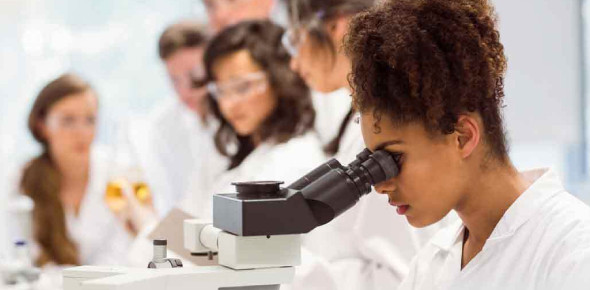# If You Pass This Biology Test, You Are A Legit Biology Enthusiast!

45 Questions | Attempts: 1596
ShareSettingsBiology is the natural science that studies life and living organisms, including their physical structure, chemical processes, molecular interactions, physiological mechanisms, development and evolution. Despite the complexity of the science, certain unifying concepts consolidate it into a single, coherent field. If you pass this biology test, you are a legit biology enthusiast!

• 1.
Organic compounds contain
• A.

Carbon and usually other elements

• B.

Many kinds of elements except carbon

• C.

Only carbon

• D.

Only carbon and hydrogen

• 2.
The number of covalent bonds a carbon atom can form with other atoms is
• A.

1

• B.

2

• C.

4

• D.

8

• 3.
A covalent bond formed when two atoms share two pairs of electrons is called a
• A.

Single bond

• B.

Double bond

• C.

Triple bond

• D.

• 4.
The breakdown of a polymer involves
• A.

Hydrolisis

• B.

A condensation reaction

• C.

The breaking of hydrogen bonds

• D.

The breaking of ionic bonds

• 5.
ATP releases energy when
• A.

It undergoes a condensation reaction

• B.

A hydroxyl group is added to it

• C.

A phosphate group is added to it

• D.

A phosphate group is removed from it

• 6.
Glycogen, starch, and cellulose are
• A.

Monosaccharides

• B.

Disaccharides

• C.

Polysaccharides

• D.

Simple sugars

• 7.
The different shapes and funtions of different proteins are determined by
• A.

The R groups of the amino acids they contain

• B.

The amino groups of the amino acids they contain

• C.

The carboxyl groups of the amino acids they contain

• D.

Whether or not they contain any amino acids

• 8.
Most enxymes
• A.

Are changed by the reactions the catalyze

• B.

Increase the activation energy of the reactions they catalyze

• C.

Strengthen the chemical bonds in their substrate

• D.

Are sensitive to changes in temperature of pH

• 9.
The large numbers of carbon hydrogen bonds in lipids
• A.

Make lipids polar

• B.

Store more energy than the carbon oxygen bonds in other organic compounds

• C.

Allow lipids to dissolve in water

• D.

Are found in the carboxyl group at the end of the lipid

• 10.
The most important function of nucleic acids is
• A.

Catalyzing chemical reactions

• B.

Forming a barrier between the inside and outside of a cell

• C.

Storing energy

• D.

Storing information related to heredity and protein synthesis

• 11.
The atomic number of carbon is 6. Therefore, the number of protons in a carbon atom equals
• A.

3

• B.

6

• C.

7

• D.

12

• 12.
One of the kinds of particles found in the nucleus of an atom is the
• A.

Proton

• B.

Electron

• C.

Ion

• D.

Boron

• 13.
The maximum number of electrons that can be held in the orbitals in an atom's second energy level is
• A.

2

• B.

4

• C.

6

• D.

8

• 14.
Of the following elements, the one that is most likely to form ionic bonds is
• A.

Hydrogen

• B.

Carbon

• C.

Sodium

• D.

Oxygen

• 15.
An example of a compound is
• A.

Water

• B.

Hydrogen gas

• C.

Oxygen gas

• D.

Chloride ion

• 16.
The state of matter in which particles move most rapidly is
• A.

Liquid

• B.

Gas

• C.

Solid

• D.

Heat

• 17.
Every chemical reaction involves a
• A.

Change in the state of the matter in the reactants

• B.

Net release of energy

• C.

Transfer of energy

• D.

Transfer of electrons between atoms

• 18.
Enzymes
• A.

Increase the amount of energy released in a reaction

• B.

Decrease the amount of energy released in a reaction

• C.

Catalyze only redox reactions

• D.

Reduce the activation energy needed for a reaction

• 19.
In chemical reactions, the number of each kind of atom in the reactants is
• A.

The same as in the products

• B.

Less than in the products

• C.

More than in the products

• D.

B or c, depending on the kind of chemical reactions

• 20.
Redox reactions
• A.

Involve either reduction of oxidation, but not both

• B.

Involve the transfer of electrons between atoms

• C.

Do not occur in living things

• D.

Always involve oxygen

• 21.
The concentration of a solution is the mesaurement of the amount of
• A.

Acid dissolved in a fixed amount of a base

• B.

Solvent dissolved in a fixed amount of the solution

• C.

Solute dissolved in a fixed amount of the solution

• D.

Solvent dissolved in a fixed amount of the solute

• 22.
When water dissociates, it forms
• A.

H+ ions and H2O

• B.

H+ ions and OH- ions

• C.

H+ ions and H3O+ ions

• D.

OH+ ions and H3O- ions

• 23.
An acid is a solution with more
• A.

Hydronium ions than hydroxide ions

• B.

Hydroxide ions than hydronium ions

• C.

Sodium ions than hydroxide ions

• D.

Hydroxide ions than sodium ions

• 24.
An example of a base is
• A.

Pure water

• B.

Vinegar

• C.

Ammonia

• D.

Urine

• 25.
A solution with a pH above 7 is
• A.

Logarithmic

• B.

Neutral

• C.

Acidic

• D.

Alkaline

## Related TopicsBack to top
×

Wait!
Here's an interesting quiz for you.